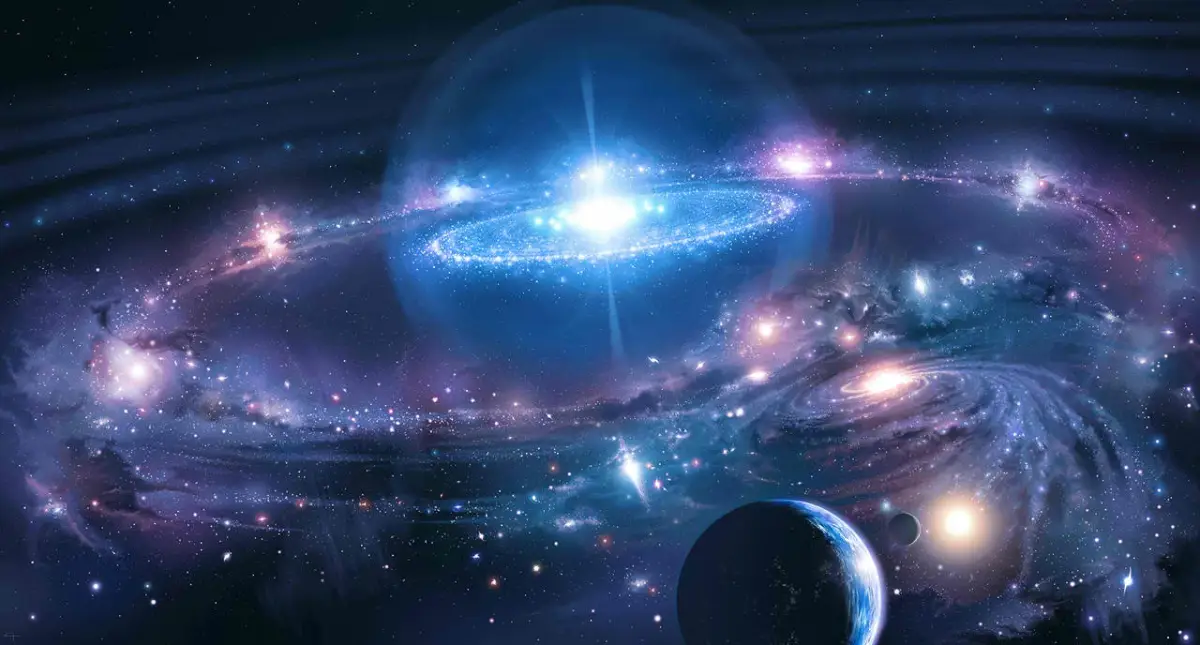## Latest Facts

### 15 Captivating Facts About Hydroponic FarmerEolande Bruner

Published: 09 Sep 2023Source: Ipilen23.wordpress.com

Newton’s Law of Universal Gravitation is one of the fundamental principles that governs the way objects interact with each other in the universe. Proposed by Sir Isaac Newton in the late 17th century, this law revolutionized our understanding of gravity and how it influences the motion of celestial bodies, as well as everyday objects on Earth. While most people have a basic understanding of gravity, there are several surprising facts about Newton’s Law of Universal Gravitation that may not be well-known. In this article, we will explore eight fascinating facts about this iconic law, shedding light on the intricacies and implications of gravity in our universe.

## Newton’s Law of Universal Gravitation Explains the Attraction Between Objects

Newton’s Law of Universal Gravitation states that every particle in the universe attracts every other particle with a force that is directly proportional to the product of their masses and inversely proportional to the square of the distance between them. This means that any two objects in the universe will exert a gravitational force on each other.

## Gravity Affects All Objects, Regardless of Their Size

Contrary to popular belief, gravity affects all objects, regardless of their size or mass. Whether it’s a small pebble or a massive planet, the force of gravity will always be present and exert its influence.

## The Apple Story is a Myth

Although the story of Isaac Newton discovering the law of gravity when an apple fell on his head is widely known, it is actually a legend. There is no historical evidence to support this popular tale, but it is often used to illustrate the concept of gravity.

## The Gravitational Force Decreases with Distance

According to Newton’s Law of Universal Gravitation, the force of gravity decreases as the distance between two objects increases. This means that the gravitational pull between objects becomes weaker as they move farther apart.

## Newton’s Law of Universal Gravitation is an Inverse Square Law

The inverse square law describes how the force of gravity weakens as the square of the distance from the source of gravity increases. This means that doubling the distance between two objects will lead to a fourfold decrease in the gravitational force.

## The Moon is Kept in Orbit by the Law of Universal Gravitation

Newton’s Law of Universal Gravitation is responsible for the moon’s orbit around the Earth. The gravitational force between the Earth and the moon keeps the moon in a stable orbit, preventing it from drifting off into space.

## Universal Gravitation Helps Explain Tides

The gravitational force between the Earth, the moon, and the sun is responsible for the occurrence of tides. The gravitational pull from these celestial bodies creates a bulge in the Earth’s oceans, resulting in the regular rise and fall of sea levels.

## Einstein’s Theory of General Relativity Expanded Upon Newton’s Law

While Newton’s Law of Universal Gravitation is incredibly accurate in most circumstances, Albert Einstein’s Theory of General Relativity provides a deeper understanding of gravity. Einstein’s theory explains gravity as the curvature of spacetime caused by massive objects.

## Conclusion

Newton’s Law of Universal Gravitation is not only a fundamental principle in physics but also a fascinating subject that offers several surprising facts. From finding out about the inverse square law to understanding the role of mass and distance, exploring the mysteries behind gravity can be an enlightening experience. Remembering that objects attract each other with a force that is directly proportional to their mass and inversely proportional to the square of the distance between them allows us to comprehend how gravity shapes our universe. By delving deeper into the complexities and intricacies of Newton’s law, we gain a greater appreciation for the profound impact it has on our daily lives and the wonders of the cosmos.

## FAQs

Q: What does Newton’s Law of Universal Gravitation state?

A: Newton’s Law of Universal Gravitation states that every particle of matter attracts every other particle with a force that is directly proportional to the product of their masses and inversely proportional to the square of the distance between them.

Q: Who discovered Newton’s Law of Universal Gravitation?

A: Sir Isaac Newton, an English physicist, mathematician, and astronomer, discovered Newton’s Law of Universal Gravitation in the late 17th century.

Q: How does mass affect the force of gravity?

A: The force of gravity is directly proportional to the mass of the objects. Increasing the mass of an object will increase its gravitational force.

Q: How does distance affect the force of gravity?

A: The force of gravity is inversely proportional to the square of the distance between two objects. As the distance between two objects increases, the gravitational force between them decreases.

Q: Is Newton’s Law of Universal Gravitation applicable only on Earth?

A: No, Newton’s Law of Universal Gravitation applies to all objects in the universe. It is a universal law that governs the gravitational interaction between any two objects, regardless of their location.

Q: Are there any exceptions to Newton’s Law of Universal Gravitation?

A: Newton’s Law of Universal Gravitation works exceptionally well in most situations. However, it does not hold true in extreme scenarios, such as in the presence of strong gravitational fields or when dealing with objects moving at speeds close to the speed of light. In such cases, Einstein’s theory of general relativity is needed to accurately describe the gravitational interaction.

Q: Can gravity be shielded or canceled?

A: Gravity cannot be shielded or canceled. It is a fundamental force that always exists and has an effect on all matter. However, its strength can be influenced by other forces, such as electromagnetic or nuclear forces, in certain circumstances.